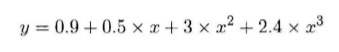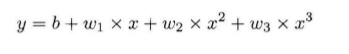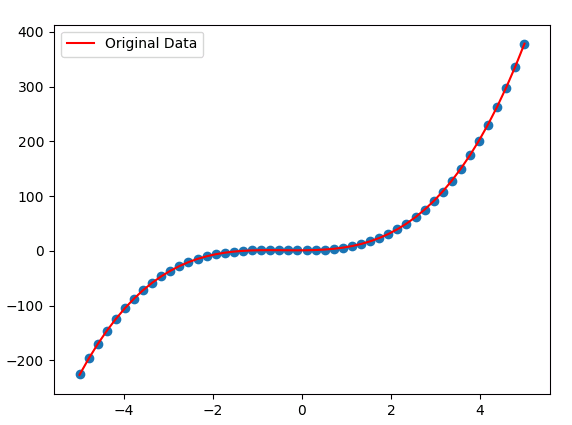``````
def make_features(x):
x = x.unsqueeze(1)

``````
def get_batch(batch_size=32):
random = torch.randn(batch_size)
x = make_features(random)
'''Compute the actual results'''
y = f(x)
if torch.cuda.is_available():
return Variable(x).cuda(), Variable(y).cuda()
else:
return Variable(x), Variable(y) ``````

``````
w_target = torch.FloatTensor([0.5,3,2.4]).unsqueeze(1)
b_target = torch.FloatTensor([0.9])

def f(x):
return x.mm(w_target)+b_target
``````

``````
class poly_model(nn.Module):
def __init__(self):
super(poly_model, self).__init__()
self.poly = nn.Linear(3,1)

def forward(self, x):
out = self.poly(x)
return out
``````
``````
if torch.cuda.is_available():
model = poly_model().cuda()
else:
model = poly_model()
``````

``````
criterion = nn.MSELoss()
optimizer = optim.SGD(model.parameters(), lr = 1e-3) ``````

``````
epoch = 0
while True:
batch_x,batch_y = get_batch()
output = model(batch_x)
loss = criterion(output,batch_y)
print_loss = loss.data
loss.backward()
optimizer.step()
epoch+=1
if print_loss < 1e-3:
break ``````

get_batch中，torch.randn是产生指定维度的随机数，如果你的机器支持GPU加速，可以将Variable放在GPU上进行运算，类似语句含义相通。

x.mm是作矩阵乘法。

nn.Linear是做一个线性的运算，参数的含义代表了输入层与输出层的结构，即3*1；在训练阶段，有几行是Pytorch不同于别的框架的，首先loss是一个Variable，通过loss.data可以取出一个Tensor，再通过data可以得到一个int或者float类型的值，我们才可以进行基本运算或者显示。每次计算梯度之前，都需要将梯度归零，否则梯度会叠加。个人觉得别的语句还是比较好懂的，如果有疑问可以在下方评论。linux命令详解date使用方法(计算母亲节和父亲节日期脚本示例)

Java枚举详解及使用实例（涵盖了所有典型用法）
log4j使用教程详解(怎么使用log4j2)
javascript Event对象详解及使用示例
Android onActivityResult和setResult方法详解及使用

Android CardView详解及使用方法和实例